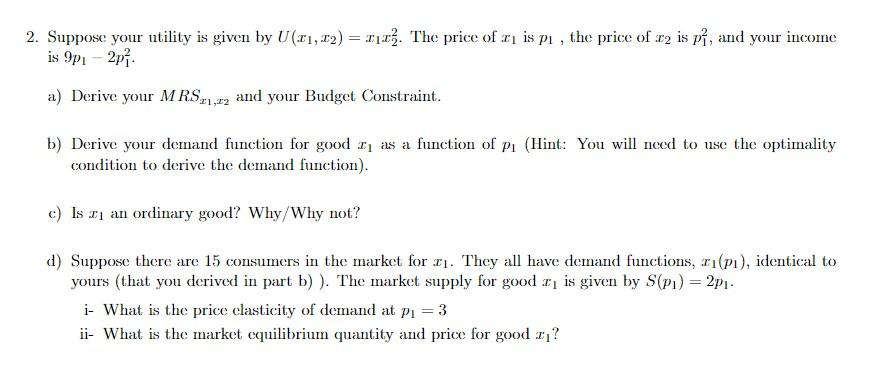### Create an Account

Home / Questions / Suppose your utility is given by U(:11, 12) = I11. The price of G is pl , the price of r2 ...

# Suppose your utility is given by U(:11, 12) = I11. The price of G is pl , the price of r2 is pî, and your income is 9p - 2p a) Derive your MRS.1,12 and your Budget Constraint. b) Derive your demand

Suppose your utility is given by U(:11, 12) = I11. The price of G is pl , the price of r2 is pî, and your income is 9p - 2p a) Derive your MRS.1,12 and your Budget Constraint. b) Derive your demand function for good 2) as a function of Pi (Hint: You will need to use the optimality condition to derive the demand function). c) Is an ordinary good? Why/Why not? d) Suppose there are 15 consumers in the market for 11. They all have demand functions, 21(P1), identical to yours (that you derived in part b) ). The market supply for good 2 is given by S(P1) = 2p1. i- What is the price clasticity of demand at pı = 3 i - What is the market equilibrium quantity and price for good I?Apr 26 2021 View more View LessSubscribe To Get Solution